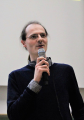Sie haben Javascript deaktiviert!
Sie haben versucht eine Funktion zu nutzen, die nur mit Javascript möglich ist. Um sämtliche Funktionalitäten unserer Internetseite zu nutzen, aktivieren Sie bitte Javascript in Ihrem Browser.

| Release

# Weierstrass Lecture 2022: Interview on the research of Prof. Dr. Peter Scholze

On Friday, 24 June, Fields Medal winner Professor Peter Scholze will be appearing as the keynote speaker at this year’s Weierstrass Lecture at Paderborn University. The mathematician is considered a genius. Professor Fabian Januszewski of Paderborn University explains what Professor Scholze’s research focuses on.

Professor Scholze specialises in arithmetic algebraic geometry – a subject that lies at the intersection of number theory and algebraic geometry. What exactly is that?

Januszewski: “Algebraic geometry is the study of the geometry solutions to polynomial equations in several variables. Usually, solutions in an algebraically closed field are examined. Number theory – i.e. arithmetic – is, in turn, the study of, among other things, solutions of such equations in the integers and in the rational numbers. Arithmetic geometry combines these two fields by studying geometric aspects of polynomial equations in several variables whose coefficients are integers or rational numbers.

In concrete terms, in arithmetic geometry, we imagine, for example, the set of prime numbers as points on an ‘arithmetic’ straight line. This enables us to obtain a geometric interpretation of the integers and prime numbers in a single geometric object. It is remarkable – and baffling – how any scientific theory can actually emerge from this.

Such an integer polynomial equation then defines not only a single geometric object, but a whole family of geometric objects – one for each prime number. In this way, arithmetic geometry unifies number-theoretic objects such as prime numbers and geometric objects from algebraic geometry in a highly sophisticated theory.”

Thanks to Professor Scholze’s work, a number of important problems that had long been considered unsolvable have now been solved. What are these problems?

Januszewski: “The first thing to say is that Peter Scholze’s contributions are primarily conceptual in nature. His work can be applied very generally, but also has a wide variety of practical applications.

Peter Scholze became known as the creator of the theory of ‘perfectoid spaces’. Perfectoid spaces – very simply – build a bridge between the geometries over different ‘p-adic’ number systems. This theory allowed him to prove special cases of mathematician Pierre Deligne’s monodromy conjecture (more specifically, the weight-monodromy conjecture) in his PhD thesis. This conjecture is significant because it allows geometric information (monodromy) to be translated into arithmetic information. He also applied perfectoid spaces to problems in the Langlands program, which consists of a network of conjectures that connect number theory, arithmetic geometry and representation theory.

In joint work with the mathematician Bhargav Bhatt, Scholze constructed the theory of ‘prismatic cohomology’, which also plays a unifying role. This theory makes it possible to build a bridge between different p-adic cohomology theories – this had been a long unsolved problem.

Together with the mathematician Dustin Clausen, Peter Scholze has laid the foundations for ‘condensed mathematics’, which can serve as a new logical basis for unifying algebra, number theory and topology, as it resolves certain incompatibilities exposed by the conventional approach.”

Scholze was awarded the Fields Medal in 2018. What was this for?

Januszewski: “Peter Scholze earned the award for transforming arithmetic algebraic geometry over p-adic fields, in particular for his theory of perfectoid spaces and their applications.”

Paderborn University was able to secure Scholze as the keynote speaker for this year’s Weierstrass Lecture. His talk will be on analytical geometry. What is his most important work in this field, and why?

Januszewski: “His major contributions here without a doubt include the introduction of perfectoid spaces, with their wide range of applications, as well as his work on integer p-adic Hodge theory and his more recent work on condensed sets. Perfectoid spaces have already revolutionised a field. The extent to which condensed sets will become established in the long term remains to be seen. Peter Scholze is undoubtedly pursuing a long-term perspective. We’re looking forward to seeing where the journey will lead in the future, and to his Weierstrass lecture!”